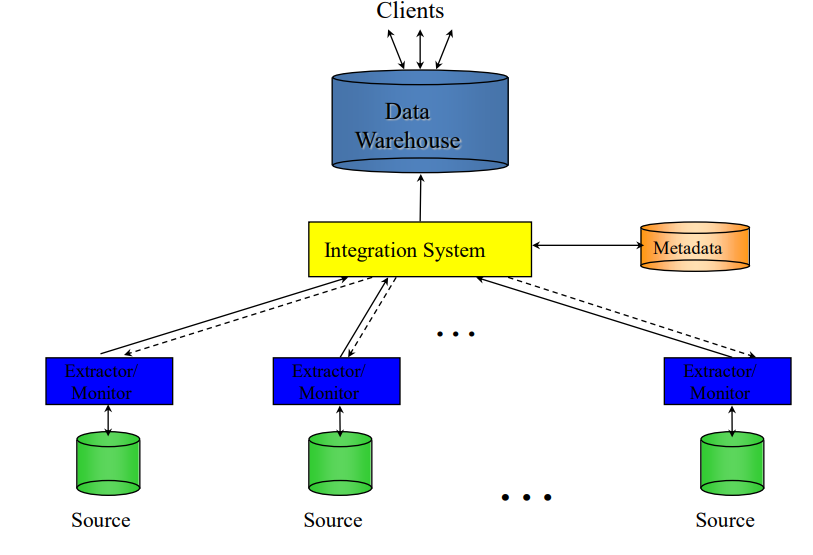# Introduction to Multidimensional Data Model

## Introduction

Data warehouses and OLAP tools are based on a multidimensional data model. Data is viewed in the form of a data cube in this approach (models n-dimensional data).The data cube is a metaphor for multidimensional data storage. Usually cubes are 3-D geometric structures, But in data warehousing the data cube is n-dimensional and do not confine data to 3-D. Data can be modelled and seen in various dimensions using a data cube. Dimensions and facts are used to define it. It’s usually organised around a central theme and displayed by a table of facts. It is commonly used in businesses to extract analytical results and generate reports that can be used as the primary source for critical decision-making processes. This paradigm is commonly used to describe systems that use Online Analytical Processing TECHNIQUES.

A simple 2-D data cube: A table or spreadsheet.Example:3-D data cube: a set of similarly structured 2-D tables stacked on top of one another. Example:

The 3-D data in the table can be represented as a series of 2-D tables called 3-D data cube, as in Figure below.

In this way, we may display any n-dimensional data as a series of (n-1)- Dimensional “cubes”.

## Working Mechanism of Multidimensional Data Model

For creating a Multidimensional Data Model,every project should go all the way through the below phases:

• Congregating the requirements from the client
• Categorising the various modules of the system.
• Spotting the various dimensions based on which the system needs to be designed.
• Drafting the real-time dimensions an the corresponding properties
• Discovering the facts from the already listed dimensionality and their properties.
• Constructing the schema to place the data,with respect to the information gathered from the above steps.

## Advantages of Multidimensional Data Model

• Its performance is superior to that of traditional databases.
• The adaptability of this type of database is a plus for projects with limited maintenance resources.
• In contrast to traditional one-dimensional database systems, it can be used on complicated systems and applications.

## Disadvantages of Multidimensional Data Model

• Because it is complicated in nature, databases are generally designed in a dynamic manner.
• Most of the time, the path to the end product is complicated.
• Because the Multi Dimensional Data Model has complicated systems and databases with a large number of databases, the system is very insecure when a security breach occurs.

## OLAP Operations in Multidimensional Data Model

The multidimensional data models are the foundation of OLAP. It enables managers and analysts to gain an understanding of the information by providing quick, consistent, and interactive access to information. Because OLAP servers are built on a multidimensional view of data, we’ll go over OLAP operations in multidimensional data. The following is a list of OLAP operations:

### Roll-up

The roll-up operation performs aggregation on a data cube, either by climbing up a concept hierarchy for a dimension or by dimension reduction

### Drill-down

Drill-down is the reverse operation of roll-up. It is performed by either by stepping down a concept hierarchy for a dimension or by introducing a new dimension. It allows users to navigate among different levels of data i.e. most summarised (up) to most details (down). Below example depicts how Drill-down operation work:### Slice

Slice operation performs a selection on one dimension of the given cube, thus creating a sub cube of a cube. Below example depicts how slice operation works- Where the sales data are selected from the central cube for the dimension time using the criterion time”Q1”.

### Dice

Dice operation performs a selection on two or more dimension from a given cube and creates a subcube. Below example depicts how Dice operation works- based on the following selection criteria: (location = “Mahendranagar” or”Dhangadi”) and (time = “Q” or “Q2”) and (item = “TV” or “PC”).

### Pivot (rotate)

Pivot also known as rotation changes the dimensional rotation of the cube, i.e. rotates the axes to view the data from different perspectives. The below cubes shows 2D representation of Pivot